# Imposed Switching Frequency Direct Torque Control of Induction Machine Using Five Level Flying Capacitors Inverter

Imposed Switching Frequency Direct Torque Control of Induction Machine Using Five Level Flying Capacitors Inverter

Department of Electrical Engineering, L2GEGI Laboratory, Ibn Khaldoun University, Tiaret, Algeria

Department of Electrical Engineering, Tahar Moulay University, Saida, Algeria

School of Engineering & Technology, University of Hertfordshire, UK

Corresponding Author Email:
abderrahmane.berkani@univ-tiaret.dz
Page:
241-248
|
DOI:
https://doi.org/10.18280/ejee.210217
27 January 2019
|
Accepted:
1 April 2019
|
Published:
30 June 2019
| Citation

OPEN ACCESS

Abstract:

The paper proposes a new control structure for sensorless induction motor drive based on a five-level voltage source inverter (VSI). The output voltages of the five-level VSI can be represented by nine groups. Then, the amplitude and the rotating velocity of the flux vector can be controlled freely. Both fast torque and optimal switching logic can be obtained. The selection is based on the value of the stator flux and the torque. This paper investigates a new control structure focused on controlling switching frequency and torque harmonics contents. These strategies, called ISFDTC, indeed combines harmoniously both these factors, without compromising the excellence of the dynamical performances typically conferred to standard DTC strategies. The validity of the proposed control technique is verified by Matlab/Simulink. Simulation results presented in this paper confirm the validity and feasibility of the proposed control approach and can be tested on experimental setup.

Keywords:

DTC, control of switching frequency, induction motor, multi-level inverter and flying capacitors inverter

1. Introduction

MUTLILEVEL inverters have received a great deal of research attention in the recent years and are nowadays widely used in high to medium voltage AC drives . Large number of output voltage levels can be produced from the multilevel converters, which brings about lower harmonic contents, lower switching loss, high voltage capability and high power quality. The principal function of multilevel converter is to synthesize a desired ac voltage from several dc voltage levels 

Generally speaking, there are three kinds of multilevel converter topologies: Diode-clamped topology, Capacitor–clamped topology and Cascade H-bridge topology .

Among these multilevel inverters, the diode-clamping inverter, which is called the neutral-point clamped (NPC) inverter, has been commonly used . However, it is difficult to control real power flow for balancing the neutral-point voltage. On the other hand, although the flying-capacitor inverter has demerits to require additional flying-capacitors and voltage control, these capacitors are smaller than DC-link capacitance and they are a simple mechanism to control charging and discharging voltage of flying-capacitors [2, 3].

There have been main three approaches to balance the voltage of dc link series capacitors in the reported papers:1) adopting separate dc sources; 2) using some auxiliary balancing circuits; 3) improving the control method by selecting redundant switching states .

Direct torque control (DTC) is the control strategies that are commonly used for variable-speed AC drives. It is characterized by the simple structure, excellent transient response and parametric robustness .

Nonetheless, classical DTC suffers from issues such as high torque and flux ripples and variable switching frequency. These demerits can be attributed mainly to three reasons: 1) the nature of varying torque and flux slopes in the motors; 2) the use of hysteresis controllers with fixed bands; 3) the availability of only a limited number of voltage vectors, which are predetermined by specific inverter topology used. Therefore, low sampling periods, leading to high switching frequencies, are often required in classical DTC to keep the torque and flux ripples to an acceptable level.

Several advanced control methods have been proposed to improve the performance of classical two-level inverter fed DTC drives. Techniques such as space vector modulation , predictive control  and duty cycle based DTC [6 of Deep] are frequently employed to improve the torque and flux regulation in the DTC drives. Both SVM-DTC and predictive-DTC are also capable of obtaining constant inverter switching frequency . Although these advanced methods contribute to alleviate the major drawbacks of the classical DTC in two Level-DTC drives, their extension to n level-DTC drives is not straightforward due to the increased degree of freedom.

In This paper the Imposed Switching Frequency Direct Torque Control (ISFDTC) strategy is generic and compatible with static converters with any number of levels. Its basic principle is applicable to different converter topologies including choppers, single-phase or three-phase inverters. However, the main application, as described in , is a multilevel three-phase voltage inverter-controlled induction motor. The ISFDTC strategy is designed to improve the quality of the supplied torque to achieve enhanced dynamics, low ripple amplitudes and harmonics frequency imposition. On the other hand, the ISFDTC strategy makes it possible to impose the average switching frequency of the inverter which leads to several advantages such as improved operation of the machine (audible noise) and the inverter and this for a wide range of torque and speed of the induction machine. Like all the control laws described as "direct", the ISFDTC acts in such a way that, at each sampling time, an instantaneous voltage vector is applied to the machine based to its current state and the performance characteristics imposed by the user. In addition, the ISFDTC strategy is based on three stages corresponding to the degrees of freedom offered by the multi-level inverters. It is well known that the number of voltage vectors at the output of a three-phase multi-level inverter increases with the number of levels of the inverter. This better meet the requirements of the regulation of the variables of the load. This degree of freedom has been classified as type I. In addition, the fact that the same voltage vector at the output of a multi-level inverter can be synthesized from several phase level sequences can be considered as a second degree of freedom which has been called type II.

Finally, a third degree of freedom for flying capacitors inverters name type III, is the ability of this type of converter to generate the same voltage level in each of its three arms with several arm configurations.

The remaining of the paper is organized as follows: Section 2 describes the model of induction machine using stationary reference frame, the operating principle and vectors generated by five level flaying capacitors inverter are presented in section 3, next section 4 describe the conventional direct torque control DTC, the three steps of proposed method of imposed switching frequency and direct torque control ISFDTC are explained in section 5, the developed method of balancing voltage of floating capacitors discussed in the section 6,  simulation results are illustrated in section 7. Finally, the concluding remarks are drawn in section 8.

2. Induction Machine Dynamics

Torque control of an induction motor can be developed using a two axis (d, q) stationary reference frame attached to the stator winding. In this reference frame, and with conventional notations, the machine electrical quantities are described by the following equations:

$\frac{d i_{s d}}{d t}=\frac{1}{\sigma T_{r} L_{s}} \varphi_{s d}+\frac{p \Omega}{\sigma L_{s}} \varphi_{s q}-\frac{1}{\sigma}\left(\frac{1}{T_{r}}+\frac{1}{T_{s}}\right) i_{s d}-p \Omega i_{s q}+\frac{1}{\sigma L_{s}} V_{s d}$                 (1)

$\frac{d i_{s q}}{d t}=\frac{1}{\sigma T_{r} L_{s}} \varphi_{s q}+\frac{p \Omega}{\sigma L_{s}} \varphi_{s d}-\frac{1}{\sigma}\left(\frac{1}{T_{r}}+\frac{1}{T_{s}}\right) i_{s q}+p \Omega i_{s d}+\frac{1}{\sigma L_{s}} V_{s q}$                 (2)

$\frac{d \varphi_{s d}}{d t}=V_{s d}-R_{s} i_{s d}$          (3)

$\frac{d \varphi_{s q}}{d t}=V_{s q}-R_{s} i_{s q}$          (4)

The mechanical equation governing to the rotor motion is described by:

$J \frac{d \Omega}{d t}=\Gamma_{e m}-\Gamma_{r}(\Omega)$         (5)

$\Gamma_{r}(\Omega) \quad$ and $\quad \Gamma_{e m}$ denote the load torque and the electromagnetic torque developed by the machine respectively.

3. Five Levels Flying Capacitors Inverter

The flying capacitors inverter structure is characterized by a nested connection of the switching cells as shown in Figure 1 . Each arm of the inverter consists of four cells.

## 1.png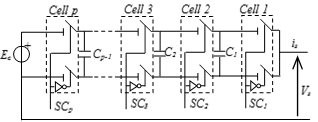Figure 1. Flying capacitors inverter arm with N-levels (p = N-1 cell)

Table 1 shows the different states of a N-levels (p-1 cells) flying capacitors converters.

Table 1. Possible states of the p-cell nested cell inverter $\Delta \mathrm{U}=E_{c} / p$

 $\mathrm{S} C_{p-1}$ … $\mathbf{S} \boldsymbol{c}_{3}$ $\mathbf{S} \boldsymbol{c}_{2}$ $\mathbf{S} \boldsymbol{c}_{1}$ $\boldsymbol{V}_{s}$ 0 … 0 0 0 0 0 … 0 0 1 $\Delta U$ 0 … 0 1 0 $\Delta U$ 0 … 0 1 1 3 $\Delta U$ 1 … 1 0 0 (p-1) $\Delta U$ 1 … 1 1 1 p $\Delta U$

The combinations of states of the three arms of the inverter allow us to generate125 vectors in total including 61 distinct vectors as shown in Figure 2.

## 2.pngFigure 2. Voltages that can be supplied by the inverter with five voltage levels

4. Direct Torque Control Strategy

Basically, DTC schemes require the estimation of the stator flux and torque. The stator flux estimation can be carried out by different techniques depending on whether the rotor angular speed or position is measured or not. For sensorless motor drive applications, the "voltage model" is usually employed . The stator flux can be evaluated by integrating the stator voltage equation.

$\varphi_{s}(t)=\int\left(V_{s}-R_{s} I_{s}\right) d t$           (6)

This method is very simple and requires the knowledge of the stator resistance only. The effect of small fluctuations in Rs is usually neglected at high switching frequencies however, as the frequency approaches zero, these effects cannot be ignored. The deviation obtained at the end of the switching period Te can be approximated by the following first order Taylor series .

$\Delta \varphi_{s} \approx V_{s} \cdot T_{e} \cdot \cos \left(\theta_{v}-\theta_{s}\right)$            (7)

$\Delta \varphi_{s} \approx T_{e} \cdot \frac{V_{s} \cdot \cos \left(\theta_{v}-\theta_{s}\right)}{\varphi_{s o}}$          (8)

Figure 3 illustrates how the adequate voltage vector is selected, to produce an increase or decrease in the stator flux amplitude and phase to achieve the desired performance.

## 3.png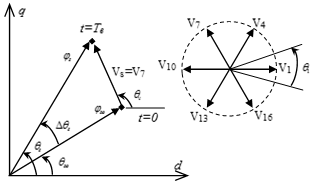Figure 3. Flux deviation in a DTC control schemeThe electromagnetic torque is estimated from the flux and current information as :

$\Gamma_{e m}=p_{p}\left(i_{s q} \varphi_{s d}-i_{s d} \varphi_{s q}\right)$         (9)

Figure 4 shows a block diagram of the DTC scheme developed by I. Takahashi . The reference values of flux, $\varphi_{s}^{*}$ , and torque $\Gamma_{e m}^{*}$ , are compared to their actual values and the resultant errors are fed into a multi-level comparator of flux and torque. The stator flux angle, $\theta_{S}$  is calculated by:

$\theta_{s}=\arctan \frac{\varphi_{s q}}{\varphi_{s d}}$         (10)

Different switching strategies can be employed to control the torque depending whether the flux is to be reduced or increased. Each strategy affects the drive behavior in terms of torque and current ripple, switching frequency and two or four quadrant operation capability. Assuming a small voltage drop $R_{s} I_{s}$, the head of the stator flux  φs  moves in the direction of stator voltage Vs at a speed proportional to the magnitude of Vs according to:

$\Delta \varphi_{s}=V_{s} T_{e}$        (11)

where $T_{e}$  is the period during which the voltage vector is applied to stator winding.

The switching configuration is applied step by step, in order to maintain the stator flux and torque within the hysteresis band. Selecting the voltage vector appropriately, it is then possible to drive  φs  along a predetermined curve .

## 4.pngFigure 4. Block diagram of ISFDTC.

Assuming that the stator flux vector lies in the kth sector (k=1,2…12) of the (d, q) plane in the case of three-level inverter. To improve the dynamic performance of DTC at low speed and to allow four-quadrant operation, it is necessary to apply the voltage vectors Vk-1 and Vk  for the control of the torque and flux.

5. Description of the Proposed Three Steps Switching Methodology

The number of vector voltages available for a three-phase voltage inverter increase with the number of levels of the inverter according to a quadratic law. In any direct control strategy, the selection of a voltage vector among all the available vectors can be considered as a degree of freedom classified as type I. This degree of freedom is the only one that can be exploited to regulate the machine variables. A second degree of freedom, classified as type II, is related to the fact that a given voltage vector can also be formed from several phase level sequences. The exploitation of this type of degree of freedom, can respond to constraints related to the static converter, or the control of the homopolar component. The flying capacitors topology allows, even within one arm, to generate a given phase voltage level, from different arm configurations. This degree of freedom, called type III, defines the current flow directions in the floating capacitors, which makes it possible, for example, to perform an active balancing of the floating capacitors. Taking these properties into account, the implementation of ISFDTC strategy can be performed into three independent steps, which can be executed in sequence as illustrated in Figure 5.

## 5.pngFigure 5. The three steps principle of ISFDTC applied to the torque of the asynchronous machine fed by a multilevel inverter

5.1 Selection of the voltage vector of the inverter

This step allows an instantaneous regulation of the torque and flux of the machine and can generalized to any multilevel inverter topology. The selection method proposed in  is applied here. For the control of the flux, the error εϕ is localized in one of the three associated intervals and which are fixed by the constraints:

$\varepsilon_{\phi}<\varepsilon_{\phi \min }$

$\varepsilon_{\phi \min } \leq \varepsilon_{\phi} \leq \varepsilon_{\phi \max }$

$\varepsilon_{\phi}>\varepsilon_{\phi \max }$         (12)

In steady-state, the electromagnetic torque is equal to the load torque. To improve the control of the torque, five control regions are defined based on the following constraints on the torque error  $\varepsilon_{T}$:

$\varepsilon_{\Gamma}<\varepsilon_{\Gamma \min 2}$

$\varepsilon_{\Gamma \min 2} \leq \varepsilon_{\Gamma} \leq \varepsilon_{\Gamma \min 1}$

$\varepsilon_{\Gamma \min 1} \leq \varepsilon_{\Gamma} \leq \varepsilon_{\Gamma \max 1}$

$\varepsilon_{\Gamma \max 1} \leq \varepsilon_{\Gamma} \leq \varepsilon_{\Gamma \max 2}$

$\varepsilon_{\Gamma \max 2}<\varepsilon_{\Gamma}$           (13)

## 6.pngFigure 6. Hysteresis control regions of the torque.

The switching table is determined based onto the outputs of the flux and torque hysteresis controllers and the flux position zone, as shown in Table 2.

Table 2. Switching table used with twelve angular sectors

Ɵ 1

 cflx ccpl 1 0 -1 6 V14 V17 V24 5 V15 V17 V25 4 V18 V17 V28 3 13 V11 V23 2 V9 V11 V19 1 V12 V11 V22 0 V0 V0 V0 -1 V52 V0 V42 -2 V56 V41 V46 -3 V53 V47 V43 -4 V58 V42 V48 -5 V55 V46 V45 -6 V54 V43 V44

Ɵ 2

 cflx ccpl 1 0 -1 6 V14 V17 V24 5 V20 V17 V30 4 V18 V17 V28 3 V13 V11 V23 2 V16 V11 V26 1 V12 V11 V22 0 V0 V0 V0 -1 V52 V0 V42 -2 V59 V41 V49 -3 V53 V47 V43 -4 V58 V42 V48 -5 V60 V49 V50 -6 V54 V43 V44

Ɵ 3

 cflx ccpl 1 0 -1 6 V24 V27 V34 5 V25 V27 V35 4 V28 V27 V38 3 V23 V21 V33 2 V19 V21 V29 1 V22 V21 V32 0 V0 V0 V0 -1 V2 V0 V52 -2 V6 V51 V56 -3 V3 V57 V53 -4 V8 V52 V58 -5 V5 V56 V55 -6 V4 V53 V54

Ɵ 4

 cflx ccpl 1 0 -1 6 V24 V27 V34 5 V30 V27 V40 4 V28 V27 V38 3 V23 V21 V33 2 V26 V21 V36 1 V22 V21 V32 0 V0 V0 V0 -1 V2 V0 V52 -2 V9 V51 V59 -3 V3 V57 V53 -4 V8 V52 V58 -5 V10 V59 V60 -6 V4 V53 V54

Ɵ 5

 cflx ccpl 1 0 -1 6 V34 V37 V44 5 V35 V37 V45 4 V38 V37 V48 3 V33 V31 V43 2 V29 V31 V39 1 V32 V31 V42 0 V0 V0 V0 -1 V12 V0 V2 -2 V16 V1 V6 -3 V13 V7 V3 -4 V18 V2 V8 -5 V15 V6 V5 -6 V14 V3 V4

Ɵ 6

 cflx ccpl 1 0 -1 6 V34 V37 V44 5 V40 V37 V50 4 V38 V37 V48 3 V33 V31 V43 2 V36 V31 V46 1 V32 V31 V42 0 V0 V0 V0 -1 V12 V0 V2 -2 V19 V1 V9 -3 V13 V7 V3 -4 V18 V2 V8 -5 V20 V9 V10 -6 V14 V3 V4

Ɵ 7

 cflx ccpl 1 0 -1 6 V44 V47 V54 5 V45 V47 V55 4 V48 V47 V58 3 V43 V41 V53 2 V39 V41 V49 1 V42 V41 V52 0 V0 V0 V0 -1 V22 V0 V12 -2 V26 V11 V16 -3 V23 V17 V13 -4 V28 V12 V18 -5 V25 V16 V15 -6 V24 V13 V14

Ɵ 8

 cflx ccpl 1 0 -1 6 V44 V47 V54 5 V50 V47 V60 4 V48 V47 V58 3 V43 V41 V53 2 V46 V41 V56 1 V42 V41 V52 0 V0 V0 V0 -1 V22 V0 V12 -2 V29 V11 V19 -3 V23 V17 V13 -4 V28 V12 V18 -5 V30 V19 V20 -6 V24 V13 V14

Ɵ 9

 cflx ccpl 1 0 -1 6 V54 V57 V4 5 V55 V57 V5 4 V58 V57 V8 3 V53 V51 V3 2 V49 V51 V59 1 V52 V51 V2 0 V0 V0 V0 -1 V32 V0 V22 -2 V36 V21 V26 -3 V33 V27 V23 -4 V38 V22 V28 -5 V35 V26 V25 -6 V34 V23 V24

Ɵ 10

 cflx ccpl 1 0 -1 6 V54 V57 V4 5 V60 V57 V10 4 V58 V57 V8 3 V53 V51 V3 2 V56 V51 V6 1 V52 V51 V2 0 V0 V0 V0 -1 V32 V0 V22 -2 V39 V21 V29 -3 V33 V27 V23 -4 V38 V22 V28 -5 V40 V29 V30 -6 V34 V23 V24

Ɵ 11

 cflx ccpl 1 0 -1 6 V4 V7 V14 5 V5 V7 V15 4 V8 V7 V18 3 V3 V1 V13 2 V59 V1 V9 1 V2 V1 V12 0 V0 V0 V0 -1 V42 V0 V32 -2 V46 V31 V36 -3 V43 V37 V33 -4 V48 V32 V38 -5 V45 V36 V35 -6 V44 V33 V34

Ɵ 12

 cflx ccpl 1 0 -1 6 V4 V7 V14 5 V10 V7 V20 4 V8 V7 V18 3 V3 V1 V13 2 V6 V1 V16 1 V2 V1 V12 0 V0 V0 V0 -1 V42 V0 V32 -2 V49 V31 V39 -3 V43 V37 V33 -4 V48 V32 V38 -5 V50 V39 V40 -6 V44 V33 V34

5.2 Selection of the phase voltage sequence

This step is also executed recursively and consists in determining, the sequence of phase levels at the next sampling period, using the degree of freedom of type II for flying capacitors inverters. It allows the balancing of commutations among the three phases.

5.3 Selection of the configuration

Once the sequence of phase levels is known, a separate phase-dependent floating capacitor balancing procedure can be used to determine the conduction states of the III cells of the three phases of the inverter, by exploiting the degrees of Type III freedom. Note that, this step is applied only for flying capacitors inverters.

6. Floating Voltage Balancing

Since both torque and stator flux of the machine are regulated by hysteresis, it was desired to extend the same method to the control of the capacitor voltages. Indeed, this method guarantees the stability of the capacitor voltages irrespective of the any variations of the reference phase levels,Cv (v=1, 2, 3, 4) which allows a total decoupling between the inverter control scheme and that of the control of the machine. The proposed rebalancing procedure is identical for all three phases and therefore the analysis is carried out for one phase only. The case of N = 5 is considered here and it is assumed that $\operatorname{sign}\left(I_{s n}\right)>0$ for all possible configurations of the inverter arm as well as the direction of evolution of each capacitor voltage. From Figure 5, the evolution rules of the following capacitor voltages can be established as follows:

(1) The configurations Conf = 0 and Conf = 15 are the only ones that make it possible to generate the levels $C_{v}^{k}=0$  and $C_{v}^{k}=4$ , respectively. In these two configurations no floating capacitor is crossed by the current and therefore the voltages will remain constant.

(2) Configurations Conf = 1, Conf = 2, Conf = 4 and Conf = 8 are used to generate the level $C_{v}^{k}=1$ . However, Conf = 1 increases Vc3, holds Vc2 and decreases Vc1; Conf = 2 hold Vc3, decrease Vc2 and increase Vc1; Conf = 4 decreases Vc3, increases Vc2 and maintains Vc1 and Conf = 8 increases Vc3, increases Vc2 and maintains Vc1.

(3) Configurations Conf = 3, Conf = 5, Conf = 6, Conf = 9 and Conf = 12 are used to generate the level $C_{v}^{k}=2$ . Conf = 3 hold Vc3, decrease Vc2 and hold Vc1; Conf = 5 decreases Vc3, increases Vc2 and decreases Vc1; Conf = 6 decreases Vc3, keeps Vc2 and increases Vc1; Conf = 9 increases Vc3, maintains Vc2 and decreases Vc1 and Conf = 12 maintains Vc3, increases Vc2 and maintains Vc1.

(3) Configurations Conf = 7, Conf = 10, Conf = 11, Conf = 13 and Conf = 14 are used to generate the level $C_{v}^{k}=3$ . Conf = 7 decreases Vc3, holds Vc2 and holds Vc1; Conf = 10 increases Vc3, decreases Vc2 and increases Vc1; Conf = 11 increases Vc3, decreases Vc2 and maintains Vc1; Conf = 13 holds Vc3, increases Vc2 and decreases Vc1 and Conf = 14 holds Vc3, holds Vc2 and increases Vc1 .

## 7.png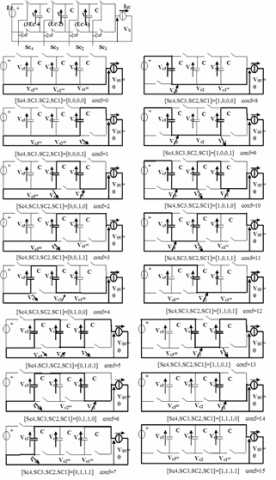Figure 7. Possible configuration of a flying capacitors inverter arm with N = 4, p = 3

## 8.png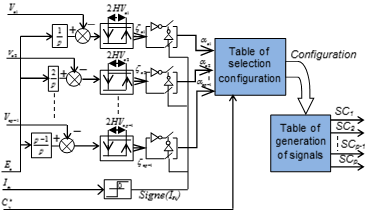Figure 8. Selection of the hysteresis balancing procedure for phase capacitor voltages

These rules were finally used to fill in the "Configuration Selection Table", shown in (Table 3), taking into account the following remarks:

$a_{c j}=0(j=1,2,3) \Rightarrow(\mathrm{Isn})>0$ therefore the voltage Vc j should not be increased.

$a_{c j}=1(j=1,2,3) \Rightarrow(\mathrm{Isn})>0$ therefore the voltage Vc j should not be decreased.

In the case where there are two possible equivalent choices $\left(a_{c 3}=0, a_{c 2}=1 a_{c 1}=0, C_{v}^{k}=1\right)$ . Indeed, in the first case it is desired, for sign (Isn)> 0, not to decrease Vc2 and not to increase Vc1 and Vc3. Figure 7 shows that both Conf = 1 and Conf = 4 achieve this requirement. However, Conf = 1 makes it possible to regulate Vc1 whereas Conf = 4 makes it possible to regulate Vc2. The first option is selected if the relative error of Vc1 is greater than that of Vc2, and the second option is selected otherwise. The same procedure is followed for the analysis of the second case $\left(a_{C 2}=0, a_{C 1}=1, C_{v}^{k}=2\right)$ .

Table 3. Configuration Selection for N = 5, p = 4

 αC3 0 0 0 0 0 0 0 0 αC2 0 0 1 1 0 0 1 1 αC1 0 1 0 1 0 1 0 1 Cp*=0 0 0 0 0 0 0 0 0 Cp*=1 1 2 1,4 4 1,8 2,8 1,8 8 Cp*=2 3 3,6 3,12 6,12 3,9 3 9,12 12 Cp*=3 7 7,14 7,13 7,14 11 10,11 13 14 Cp*=4 15 15 15 15 15 15 15 15
7. Simulation Results

In this subsection, the transient and steady state performances of the proposed algorithm ISFDTC are compared to the conventional DTC method in , which is referred to as CDTCM in the continuation of this paper for simplicity. In the simulations, both classical DTC method and the proposed ISFDTC operate with a sampling frequency of 20 kHz. The torque regulator in the proposed ISFDTC operates with a imposed frequency $\left(f_{i}\right)$  to 1.25 kHz $f_{i}=\frac{f_{s}}{4 \rho}$  where $\rho$ : number of cell and $f_{s}$  is sampling frequency , while the average inverter switching frequency of classical DTC method is variable, with a mean value of approximately 1 to 20 kHz Figure 9.

The ISFDTC strategy is applied to direct torque control of an induction machine driven by a 5-level inverter.

Figure 10 shows the waveforms of the main quantities of the induction machine controlled by the ISFDTC strategy compared to the direct torque control with conventional method where the waveforms are shows in Figure 9. It can be observed that the torque and stator flux are successfully controlled within their respective hysteresis band. The amplitude of the flux vector increases then remains constant and equal to its reference value with a low ripple. This demonstrates a good regulation of the flux and effective decoupling with the torque during the transient conditions. The waveforms of the steady-state capacitor voltages are shown in Figure 10. Hence, it can be confirmed by simulation that, independently of the phase voltage level, it is always possible to select an arm configuration that makes it possible to confine the evolution of the capacitor voltages within their hysteresis band. Figure 10(e) shows the voltages at the terminals of each floating capacitor. All capacitor voltages converge to their respective set points. The average switching frequency ( $f_{i}=1.25 k H z$ ) is practically constant. It is important to remember that this ability to correctly impose the switching frequency is a major advantage of the ISFDTC strategy that did was not found in conventional DTC strategies.

## 9.png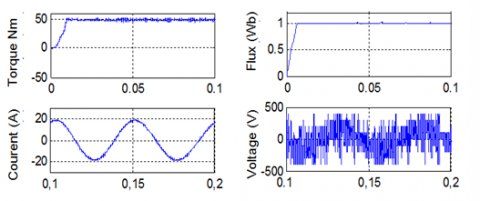Figure 9. Response of conventional DTC: torque, flux, stator current, voltage

## 10.pngFigure 10. Response of ISFDTC torque, flux, stator current, voltage, evolution of voltages at floating capacitor terminals, and average switching frequency per cell

8. Conclusions

This paper focused on the application ISFDTC principle to direct torque control of an induction machine driven by a five-level multicell inverter. The control design was divided into three stages, each of which allows us to exploit a different type of degree of freedom:

1. The first step is devoted to the selection of the inverter voltage vector, contributing to the adjustment of the torque and stator flux.
2. The second step consists of adjusting the homopolar component of the voltage supplied by the inverter, so as to select the sequence of phase levels that best contributes to the equalization of the number of switches among the three phases of the inverter, thus contributing to impose the average switching frequency.
3. The third step generates the physical control signals of the converter cells, allowing the balancing of the voltages at the terminals of the floating capacitors.

The simulation results show good stability and improved control of the induction motor drive. Thus, the algorithm proposed for the direct control of the torque of the asynchronous machine powered by a 5-level inverter achieves good dynamic and static performance. We conclude that this control method provides better steady-state results compared to the conventional method.

Nomenclature
 IM ISFDTC Induction Motor Imposed Switching Frequency Direct Torque Control Г Electromagnetic Torque φ $\theta_{s}$ Flux linkage The stator flux angle Isv V εГ, εφ Current Voltage Error torque and flux respectively fs Sampling frequency Te Sampling time fi Imposed frequency Ec DC voltage link p Number of cell of inverter Vc Voltage capacitor αc Configuration of converter arm
Appendix

Table 4. Parameters of the induction motor used in the simulations

 type Three-phase induction machine Power 7.457 kW Nominal voltage 460 V Nominal speed 1760 rpm/min Rated frequency 60 Hz Stator resistance 0.6837 ohm Rotor resistance 0.451 ohm Stator inductance 0.004152 H Rotor inductance 0.004152 H Mutual inductance 0.1486 H Number of pole pairs pp=2 Moment of inertia 0.05 Nms2/rad Coefficient of friction 0.008141 Nms/rad
References

 Mohan, D., Zhang, X., Gilbert, F. (2017). Generalized DTC strategy for multilevel inverter fed IPMSMs with constant inverter switching frequency and reduced torque ripples. IEEE Transactions on Energy Conversion, 32(3): 1031-1041. https://doi.org/10.1109/TEC.2017.2681653

 He, L.Z., Chen C. (2016). Flying-capacitor-clamped five-level inverter based on bridge modular switched-capacitor topology. IEEE Transactions on Industrial Electronics, 63(12): 7814-7822. https://doi.org/10.1109/TIE.2016.2607155

 Chen, C., He, L.Z., (2016). Flying-capacitor-clamped five-level inverter based on switched-capacitor topology. IEEE Energy Conversion Congress and Exposition (ECCE). https://doi.org/10.1109/ECCE.2016.7855123

 Park, D.H., Ku, N.J., Kim, R.Y. (2015). A novel switching loss minimization method for single-phase flying-capacitor multilevel inverter. IEEE 2nd International Future Energy Electronics Conference (IFEEC). https://doi.org/10.1109/IFEEC.2015.7361592.

 Vafaie, M.H., Dehkordi, B.M., Moallem, P., Kiyoumarsi, A. (2016). Minimizing torque and flux ripples and improving dynamic response of PMSM using a voltage vector with optimal parameters. IEEE Trans.Ind. Electron, 63(6): 3876-3888. https://doi.org/10.1109/TIE.2015.2497251

 Martins, C.A., Meynard, T.A., Roboam, X., Carvalho, A.S. (1999). A predictive sampling scale model for direct torque control of the induction machine fed by multilevel voltage-source inverters. The European Physical Journal - Applied Physics, 5(1): 51-61. https://doi.org/10.1051/epjap:1999111

 Ammar, A., Kheldoun, A., Metidji, B., Talbi, B., Ameid, T., Azzoug, Y., (2018). An experimental assessment of direct torque control and model predictive control methods for induction machine drive. International Conference on Electrical Sciences and Technologies in Maghreb (CISTEM). https://doi.org/10.1109/CISTEM.2018.8613419

 Zaimeddine, R., Refoufi, L., Berkouk, E.M. (2007). An improved direct torque control strategy for induction motor drive. International Journal of Electrical and Power Engineering. (IJEPE), 1(1): 21-27. http://medwelljournals.com/abstract/?doi=ijepe.2007.21.27

 Mohd, A.I., Beum, L.K. (2017). Modified frequency carriers for improving DTC with constant frequency torque controller of induction machines. IEEE Conference on Energy Conversion (CENCON). https://doi.org/10.1109/CENCON.2017.8262471

 Azura A.T.S., Jidin, A., Huzainirah, I., Khairi, R.M., Abdul, K.K. (2016). DTC brushless DC motor by using constant switching frequency. IEEE International Conference on Power and Energy (PECon). https://doi.org/10.1109/PECON.2016.7951560

 Chattopadhyay, S.K., Chakraborty, C., (2017). A new technique for capacitor balancing of three-level flying-capacitor multilevel inverter. IECON 2017 - 43rd Annual Conference of the IEEE Industrial Electronics Society. https://doi.org/10.1109/IECON.2017.8217107

 Rodrigo, Moritz, M.B., Batschauer, A.L. (2017). Capacitor voltage balancing in a 5-L full-bridge flying capacitor inverter. Brazilian Power Electronics Conference (COBEP). https://doi.org/10.1109/COBEP.2017.8257234